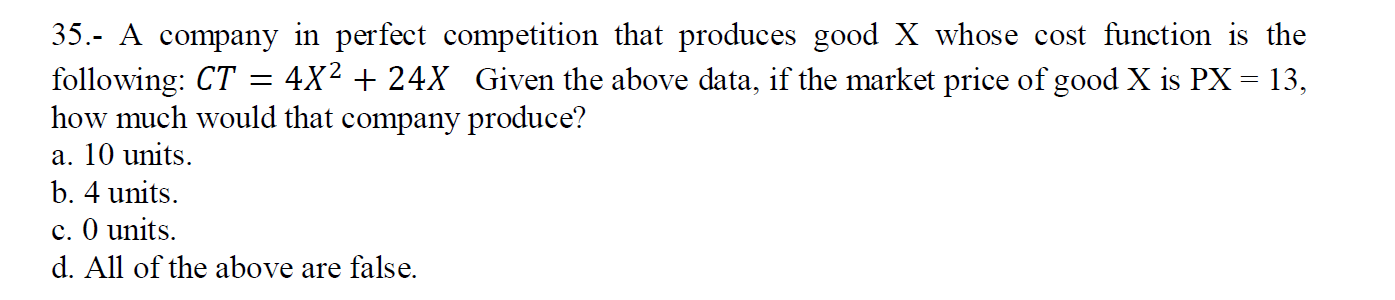Home / Expert Answers / Economics / 35-a-company-in-perfect-competition-that-produces-good-x-whose-cost-function-is-the-follow-pa776

# (Solved): 35.- A company in perfect competition that produces good $$X$$ whose cost function is the follow ...35.- A company in perfect competition that produces good $$X$$ whose cost function is the following: $$C T=4 X^{2}+24 X$$ Given the above data, if the market price of good $$\mathrm{X}$$ is $$\mathrm{PX}=13$$, how much would that company produce? a. 10 units. b. 4 units. c. 0 units. d. All of the above are false.

We have an Answer from Expert